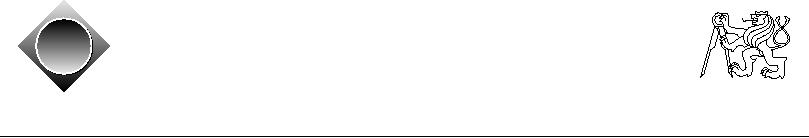Czech ACM Student Chapter
Czech Technical University in Prague
Charles University in Prague
Technical University of Ostrava
acm
ˇ a
Slovak University of Technology
Pavol Jozef Saf´rik University in Koˇice
s
cz
ˇ
University of Zilina
Masaryk University
Matej Bel University in Bansk´ Bystrica
a
University of West Bohemia
CTU Open Contest 2011
Ambiguous Result
result.c, result.C, result.java, result.p
The ACM (Advanced Cosmos Monitor) recorded a set of messages transmitted by alien race of
Space Invaders. Unfortunately, the antenna used for recording only handles lower frequencies
representing numbers and two arithmetical operators in space-invaderian language, while all
parentheses (corresponding to a high frequency) were lost.
Since numbers are important for those 8-bit creatures, we really need to know what number
ranges these messages belong to -- please, write a program that can do this for us!
Input Specification
Input contains several legal arithmetical expressions, each expression on a separate line. Each
expression consists only of non-negative integers xi (0 xi 100) and binary operators "+"
and "*". The expression starts with a number, then the operators and numbers alternate, and
the last element is a number. Each expression contains P numbers (1 P 100) and P - 1
operators. There are no parentheses, no other operators, no unary operator, etc.
The last input expression is followed by a line containing the single word "END".
Output Specification
For each input line (not counting the final END), output one line containing the minimum and
maximum values (separated by a single space) that are achievable by adding parentheses to the
input in a way that forms a legal expression and computing the result of that expression.
For example, the minimum value for 2 + 1 0 input is achieved by (2 + 1) 0 and the maximum
value is achieved by 2 + (1 0). Therefore, you should print "0 2".
It is guaranteed that for any placement of parentheses, the value of each parenthesis will be less
then 263. This means that also the maximal result will be between 0 and 263 - 1, inclusive.
Sample Input
Output for Sample Input
2+1*0
02
3+2*5+1*7+16
36 690
0
00
END